### LOCATING GEOSYNCHRONOUS SATELLITES

INTRODUCTION

 Geosynchronous satellites are those that appear to remain nearly stationary in the sky as observed from a point on the Earth's surface. They do so because their period of revolution about the Earth is identical to the period of rotation of the Earth about its polar axis. A true geostationary satellite will remain absolutely fixed at the same point in the sky as seen by a ground observer. However, perturbing forces such as radiation pressure, Earth non-sphericity and lunar and solar gravitational forces, all conspire to remove a satellite from a true geostationary orbit. Ground directed manuevres are required to counteract these forces and maintain the satellite in what is really a geosynchronous orbit - an orbit that is close to but not exactly geostationary. These corrections are termed station keeping, and are carried out to keep the satellite in a virtual box around the true geostationary point. This box usually has dimensions of less than one degree of arc, as viewed from the ground.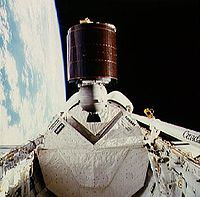Australia's first geosynchronous satellite, AUSSAT-A1 being deployed from the payload bay of Space Shuttle mission STS-51I.(NASA image)

Station keeping has several objectives. If the satellite is used to broadcast TV or data, it means that small receiving antennae do not need to be moved to track the satellite. It also helps minimise interference between adjacent satellites using the same frequency band. And it is one step toward minimising collisions between active satellites.

Sometimes, if the station keeping fuel on board a geosat becomes low, the inclination of the satellite (the angle that its orbital plane makes with the Earth's equator) is allowed to increase from zero. Such satellites show a figure of eight motion in the sky. The satellite sweeps around the figure of eight with a period of 24 hours.

Thus a ground observer is likely to see a combination of secular drift, periodic motions (eg figure of eight) and abrupt correctional changes (due to thruster firings) in the motion of a geosynchronous satellite. However, these are usually small, and for many locational purposes it is adequate to compute look angles assuming the simplicity of geostationarity.

SPECIFICATION OF A GEOSTATIONARY ORBIT

A geostationary orbit has specific orbital parameters. It is first of all a circular orbit. This means that it has an eccentricity of zero - its distance from the centre of the Earth is always the same (42,165 km). It furthermore has an orbital inclination of zero - its orbital plane is identical to the Earth's equatorial plane. Lastly, it has a period (related to its orbital radius) of 86164 seconds (about 23 hours 56 minutes 4 seconds), which is the time it takes the Earth to rotate through 360 degrees.

The location of a satellite is usually computed using an ephemeris program and a set of orbital elements. However, for a stationary geosat, this is unnecessary and its position may be computed using only geometry. The only parameter needed to specify a geosat is its longitude. This is the same as the longitude of a point on the Earth's equator directly below the satellite itself.

Geosat longitude may be specified from 0 to 360 degrees, measured eastward around the equator from the zero degree meridian passing through Greenwich, England. Alternatively it might be specified from 0 to +180 degrees (east) and 0 to -180 degrees (west). Note that +180 and -180 are the same point.

GEOSAT GEOMETRY

We need both plane trigonometry and spherical trigonometry to derive the equations needed to compute geostationary satellite look angles.

We start with spherical trigonmetry. This will give us the azimuth angle we require and also specify the plane in which we use plane trigonometry to find the satellite elevation.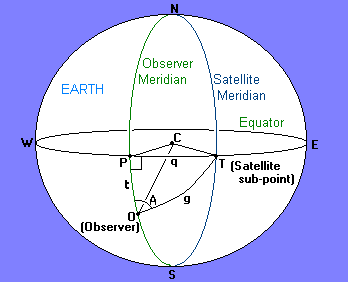Spherical geometry for geosat location.

The above diagram shows points on the surface of the Earth. O is the observer, C is the centre of the Earth. P is a point on the equator with the same longitude as the observer, and T is the point directly below the satellite. All curved lines on the diagram are parts of great circles.

We are interested in the spherical triangle OPT. The 'distance' t is the latitude of the observer, q is the longitudinal difference between the observer and the satellite (or sub-satellite point), and g is the great circle distance from the observer to the sub-satellite point. The azimuth angle A is the angle measured from north of the satellite, and this is the angle we are trying to calculate.

The sides of the spherical triangle are related by:

cos(g) = cos(q) cos(t)
Since we know the angles t and q, we can calculate the angle g. Knowing this we can then calculate the azimuth from the equation:
sin(A) = sin(q) / sin(g)
We then move to consider a plane triangle in the plane OTC which contains both the observer and the satellite.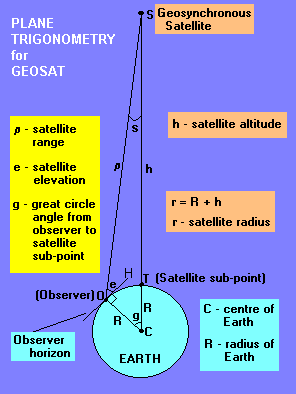Plane geometry for geosat location

In the triangle COS, the satellite elevation is given by:

tan(e) = [(R+h)cos(g) - R] / [(R+h)sin(g)]
and the slant range to the satellite can be found from:
ρ = sqrt [ R2 + (R+h)2 - 2 R(R+h)cos(g) ]
To complete our calculations we need only to know that R=6378 km and r=(R+h)= 42165 km, which are the equatorial radius of the Earth and the satellite orbital radius respectively.

These formula assume a perfectly spherical Earth, whereas it is in fact an oblate spheroid with the polar radius less than the above quoted equatorial radius. Calculations with an oblate Earth model show that the errors incurred from the spherical approximation amount to less than 0.1 degree in azimuth and elevation and 10 km in slant range, at mid-latitudes. This error is less than many station keeping regimes.

GEOSAT LOOK ANGLE CHARTS

The above formula can be used to plot look angle charts. These show both azimuth and elevation angles as a function of observer latitude and longitudinal difference from the satellite.

The chart below is the one to use at mid-latitudes.The azimuth contours are shown in white or red and are plotted every 2 degrees. The elevation contours are also plotted every 2 degrees in blue or cyan. The chart can thus be read to an accuracy of one degree with ease.

The azimuth angles shown in the above chart are in the range from 0 to 90 degrees. The true azimuth is computed from these values according to the quadrant in which the observer lies with respect to the satellite. The modification formulae are shown in the quadrant diagram below.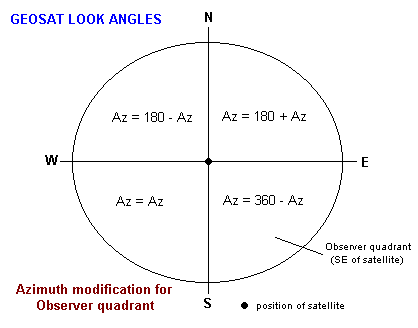As an example, consider an observer at a latitude of 40 degrees north and 32 degrees to the east of the desired satellite. From the look angle chart we find an elevation of 34 degrees and an azimuth of 44 degrees. However, as the observer is in the quadrant to the north-east of the satellite, the true azimuth is 180+44 or 224 degrees.

For observers in the tropics the look angle chart becomes very congested, and an equatorial supplement is provided below.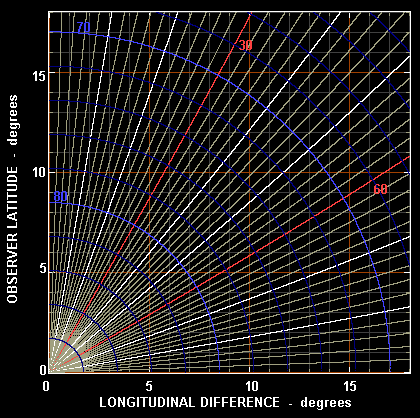Note that a geosynchronous satellite is below the horizon when the great circle distance to its sub-point exceeds 81 degrees. This means no geosynchronous satellite coverage in the polar regions. Geosat communication is possible around the coastline of Antarctica but necessitates very large ground antennae (eg 20 metre class dishes).

EQUATORIAL/POLAR COORDINATES

For astronomical and some other purposes, it is more convenient to have the coordinates of a geosynchronous satellite in terms of declination(δ) and hour angle(HA). These may be computed from elevation and azimuth according to the formula:

sin(δ) = cos(A)cos(t)cos(e) + sin(t)sin(e)

cos(HA) = ( sin(e) - sin(t)sin(δ) ) / ( cos(t)cos(δ) )

Note that δ is positive to the north of the equatorial plane and negative to the south. The hour angle (HA) is negative in the east and positive in the west.

The above two equatorial coordinates are constant because the satellite is fixed in the sky. However, the stars are not fixed and accurate positions are often derived from stellar position comparison. For this purpose it is necessary to change the hour angle coordinate into right ascension. This is done using the formula:

RA = LST - HA
where LST is the local sidereal time at the observing location.

Note that RA, LST and HA must all be in the same units, which is usually hours, but may also be degrees. Note also that the right ascension of a geosat is continuously changing, and if this coordinate is specified it is vital to specify the time of measurement.

The following plot shows how the declination of a satellite changes with longitudinal difference.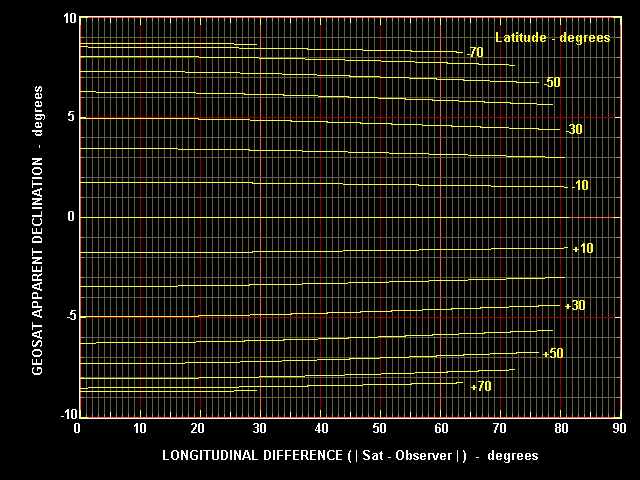The declination of a geosat will always lie between + and - 9 degrees. For mid-latitudes the declination is around -5 degrees in the northern hemisphere and +5 degrees in the southern hemisphere. For a given location the declination of any visible geosat never varies by more than about half a degree.

The band in the sky across which geostationary satellites are found is sometimes referred to as the Clarke Belt, a tribute to the writer Arthur C Clarke who first suggested the use of this orbit in 1945. The Clarke Belt is shown for the Australian Space Academy site in the diagram below. Several satellites are shown along the belt.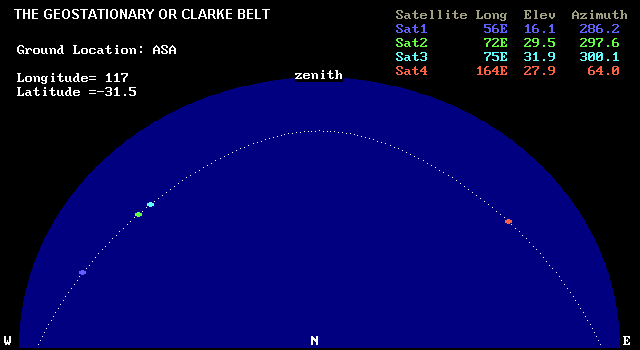PROGRAMMING THE FORMULA

Coding the above formula into a computer program is relatively straight forward. The main difficulty usually arises in putting things in their correct quadrant. A simple QBASIC program that produces geosat coordinates in both the horizon coordinate system (elevation-azimuth) and the equatorial coordinate system (declination-hour angle) is given below.

```'GEOSATL.BAS
CLS
PRINT " GEOSTATIONARY SATELLITE LOOK ANGLES"
ER = 6378: HS = 35786!                    'earth radius / satellite ht
pi = 3.14159: dr = pi / 180               'pi /degrees to radians const
lon = 117: lat = -32                      'ground station long and lat (ASA)
ilon = CINT(lon)                          'round longitude to nearest integer
GT = lat * dr                             'convert ground lat to radians
form\$ = "      ####.#      ##.#      ###.#   ##.###  ###.##    #####   #####"
PRINT USING "   Observer LAT=###.#  LON=####.# "; lat; lon
PRINT
PRINT "     SAT-LONG   ELEVANGLE  AZANGLE    HA      DEC     SLANT    GND"
PRINT "       (deg)      (deg)     (deg)    (hrs)   (deg)     (km)    (km)"
FOR SN = ilon - 85 TO ilon + 85 STEP 10  'only sats. within 81.3deg vis
B = (lon - SN) * dr                    'longitude diff between sat & gnd
GC = COS(B) * COS(GT)                  'GC is the great circle angle
GC = ATN(SQR(1 - GC * GC) / GC)        'between gnd station and satellite
IF GC < 1.419 THEN                     'if greater than 81.3deg skip
gr = ER * GC                         'ground range to sat sub point
az = ATN(TAN(B) / SIN(GT))           'compute azimuth angle
IF GT >= 0 THEN az = az + pi         'adjust for northern hemisphere
az = az - INT(az / 2 / pi) * 2 * pi  'ensure lies tween 0 and 360deg
sr = SQR(ER ^ 2 + (ER + HS) ^ 2 - 2 * ER * (ER + HS) * COS(GC)) 'slant range to sat
ZA = ((ER + HS) ^ 2 - sr ^ 2 - ER ^ 2) / 2 / ER / sr  'compute zenith angle
ZA = ATN(SQR(ABS(1 - ZA * ZA)) / ZA): el = pi / 2 - ZA  'of satellite then elevation
DC = COS(az) * COS(GT) * SIN(ZA) + SIN(GT) * COS(ZA) 'compute declination
DC = ATN(DC / SQR(1 - DC * DC))                 'with simulated ASIN func
HA = (COS(ZA) - SIN(GT) * SIN(DC)) / COS(GT) / COS(DC) 'compute hour angle of sat
HA = ATN(SQR(1 - HA * HA) / HA)               'with simulated ACOS func
IF az < pi THEN HA = -HA                      'take care of sign
HA = HA / dr / 15
S = SN + 180: S = S - INT(S / 360) * 360 - 180  'sat lon to lie -180 to 180
PRINT USING form\$; S; el / dr; az / dr; HA; DC / dr; sr; gr
END IF
NEXT SN
END
```

A typical output is displayed below.

 ``` GEOSTATIONARY SATELLITE LOOK ANGLES Observer LAT=-32.0 LON= 117.0 SAT-LONG ELEVANGLE AZANGLE HA DEC SLANT GND (deg) (deg) (deg) (hrs) (deg) (km) (km) 42.0 4.0 278.1 5.487 4.70 41236 8607 52.0 12.5 283.9 4.801 4.81 40320 7681 62.0 21.0 290.4 4.098 4.91 39457 6779 72.0 29.3 297.9 3.380 5.01 38678 5917 82.0 37.1 307.1 2.647 5.10 38011 5120 92.0 44.0 318.7 1.901 5.17 37485 4427 102.0 49.3 333.2 1.145 5.22 37120 3896 112.0 52.4 350.6 0.382 5.25 36934 3601 122.0 52.4 9.4 -0.382 5.25 36934 3601 132.0 49.3 26.8 -1.145 5.22 37120 3896 142.0 44.0 41.3 -1.901 5.17 37485 4427 152.0 37.1 52.9 -2.647 5.10 38011 5120 162.0 29.3 62.1 -3.380 5.01 38678 5917 172.0 21.0 69.6 -4.098 4.91 39457 6779 -178.0 12.5 76.1 -4.801 4.81 40320 7681 -168.0 4.0 81.9 -5.487 4.70 41236 8607 ```

The last column in the above table is the great circle distance from the observer to the satellite sub-point.Australian Space Academy